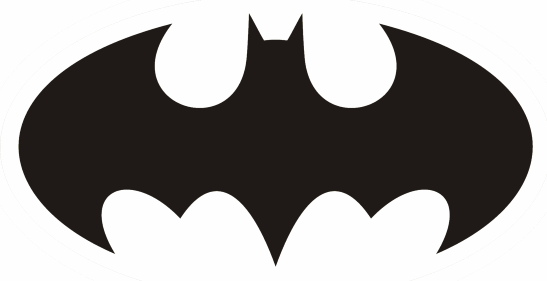# Mickey Mouse, Batman, and conformal mapping

A conformal map between two regions in the plane preserves angles . If two curves meet at a given angle in the domain, their images will meet at the same angle in the range. Two subsets of the plane are conformally equivalent if there is a conformal map between them.

The Riemann mapping theorem says that any simply connected proper open subset of the plane is conformally equivalent to the interior of the unit disk. Simply connected means that the set has no holes . Proper means that the set is not the entire plane .

Two regions that are conformally equivalent to the disk are conformally equivalent to each other. That means if you have a region like a Mickey Mouse silhouetteand another region like the Batman logothen there’s a conformal map between them. If you specify a point in the domain and where it goes in the range, and you specify its derivative at that point, then the conformal map is unique.

Now this only applies to open sets, so the boundary of the figures is not included. The boundaries of the figures above clearly have different angles, so it wouldn’t be possible to extend the conformal map to the boundaries. But move in from the boundary by any positive distance and the map will be conformal.

Conformal maps are useful transformations in applications because they’re very nice functions to work with. In theory, you could write out a function that maps Mickey Mouse to Batman and one that goes the other way around. These functions would be difficult if not impossible to write down analytically, but there is software to find conformal maps numerically. For simple geometric shapes there are reference books that give exact conformal maps.

The Riemann mapping theorem applies to regions with no holes. What if we take two regions that do have a hole in them? To keep things simple, we will look at two annular regions, that is, each is the region between two circles. For i = 1 and 2 let Ai be the region between two circles centered at the origin, with inner radius ri and outer radius Ri. Are these regions conformally equivalent?

Clearly they are if R1/r1 = R2/r2, i.e. if one annulus is a magnification of the other. In that case the conformal map is given by mapping z to (r2/r1) z.

Here’s a big surprise: this is the only way the two annuli can be conformally equivalent! That is, if R1/r1 does not equal R2/r2 then there is no conformal map between the two regions.

So suppose you have to rings, both with inner radius 1. One has outer radius 2 and the other has outer radius 3. Then these rings are not conformally equivalent. They look a lot more like each other than do Mickey Mouse and Batman, but the latter are conformally equivalent and the former are not.

Almost any two regions without holes are conformally equivalent, but it’s much harder for two regions with holes to be conformally equivalent.

## Related posts

 Conformal maps also preserve orientation. So if you move clockwise from the first curve to the second in the domain, you move clockwise from the image of the first to the image of the second by the same angle.

A function of a complex variable is conformal if and only if it is holomorphic, i.e. analytic on the interior of its domain, with non-zero derivative. Some authors leave out the orientation preserving part of the definition; under their weaker definition a conformal map could also be the conjugate of a holomophic function.

 A rigorous way to say that a region has no holes is to say its fundamental group is trivial. Any loop inside the region is homotopic to a point, i.e. you can continuously shrink it to a point without leaving the region.

 Liouville’s theorem says an analytic function that is bounded on the entire plane must be constant. That’s why the Riemann mapping theorem says a region must be a proper subset of the plane, not the whole plane.